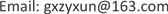GSTGeomatics Science and Technology2329-549XScientific Research Publishing10.12677/GST.2019.72017GST-29912GST20190200000_98653866.pdf工程技术 几种世界地图投影的经纬网的数学变换 Mathematical Transformation of Latitude and Longitude Network on Several World Map Projections 业勋1*佳奇32宝清42南宁师范大学，北部湾环境演变与资源利用省部共建教育部重点实验室，广西 南宁；南宁师范大学，广西地表过程与智能模拟重点实验室，广西 南宁海军工程大学导航工程系，湖北 武汉；南宁师范大学，北部湾环境演变与资源利用省部共建教育部重点实验室，广西 南宁；南宁师范大学，广西地表过程与智能模拟重点实验室，广西 南宁；广西测绘地理信息局，广西 南宁海军工程大学导航工程系，湖北 武汉null180320190702117123© Copyright 2014 by authors and Scientific Research Publishing Inc. 2014This work is licensed under the Creative Commons Attribution International License (CC BY). http://creativecommons.org/licenses/by/4.0/

1海军工程大学导航工程系，湖北 武汉

2南宁师范大学，北部湾环境演变与资源利用省部共建教育部重点实验室，广西 南宁

3南宁师范大学，广西地表过程与智能模拟重点实验室，广西 南宁

4广西测绘地理信息局，广西 南宁1. 引言

2. 合同图形

M s 0 : x ′ = − x ∧ y ′ = y (2)

3. 用于世界地图的正圆柱投影、伪圆柱投影和多圆锥投影的经纬网

M λ 0 ∈ D φ λ a d ∨ D φ λ b d ∨ D φ λ b c ∧ M s 0 ∈ D φ λ a d ∨ D φ λ b d ∨ D φ λ b c (3)

∃ λ 0 ∈ D φ λ ⇒ ∃ λ i ∉ λ 0 ∧ λ i ′ ∉ λ 0 ∧ λ i ∩ φ j = M i j , λ i ′ ∩ φ j = M i ′ j ⇒ λ 0 ⊥ M i j M i ′ j (4)

3.1. 外凸型曲经线族

D λ 0 = { λ i | ∀ λ i ∉ λ 0 ∧ τ i > 0 ∧ T i > 0 , i ∈ D I } (5)

3.2. 离散型直经线族

D λ * = { λ i | ∀ λ i ∉ λ 0 ∧ τ i = 0 ∧ T i = ∞ , i ∈ D I } (6)

3.3. 内凸同轴圆弧型纬线族

D φ 0 = { φ j | φ j ∉ s 0 ∧ φ j ∈ 2 π R j , R j = g ( φ j ) = Q j M j 0 , j ∈ D J } (7)

(7)式表明，非零纬线为同轴( λ 0 )圆弧，圆心为 Q j ，半径为 R j ，它们是凸部指向赤道的曲线族。

3.4. 赤道平行线型纬线族

D φ d = { φ j d | ∀ φ j d ∉ s 0 ⇒ R j d = ∞ ∈ φ j d , j ∈ D J } (8)

(8)式表明，非零纬线均为半径为无穷大的圆弧，也就是直线。于是， D φ d ∪ s 0 = D φ ，这是赤道平行线型纬线网，又可表达为 D φ = { φ j d ∥ s 0 | φ j d ∉ s 0 } ，记作

4. 正圆柱投影、伪圆柱投影和多圆锥投影的构成和投影变换

4.1. 正圆柱投影

D φ λ a d = D λ a ∪ D φ d (9)

4.2. 伪圆柱投影

D φ λ b d = D λ b ∪ D φ d (10)

4.3. 多圆锥投影

D φ λ b c = D λ b ∪ D φ c (11)

4.4. 多圆锥投影–伪圆柱投影变换

( D φ λ b c / D φ c ) ∪ D φ d = D φ λ b d (12)

4.5. 伪圆柱投影–圆柱投影变换

( D φ λ b d / D λ b ) ∪ D φ a = D φ λ a d (13)

4.6. 伪圆柱投影–多圆锥投影变换

( D φ λ b d / D φ d ) ∪ D φ c = D φ λ b c (14)

( D φ λ b d ∩ ∅ ) ∪ D φ λ b c = D φ λ b c (15)

5. 结论